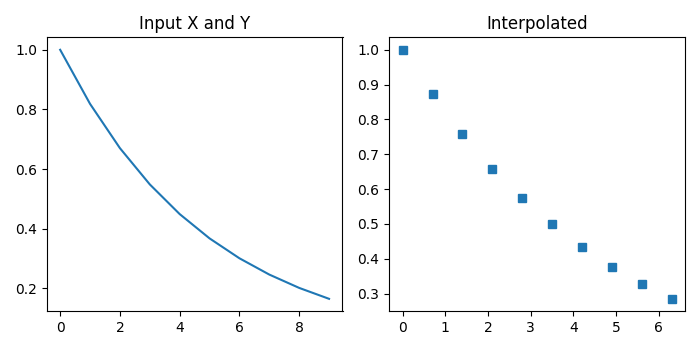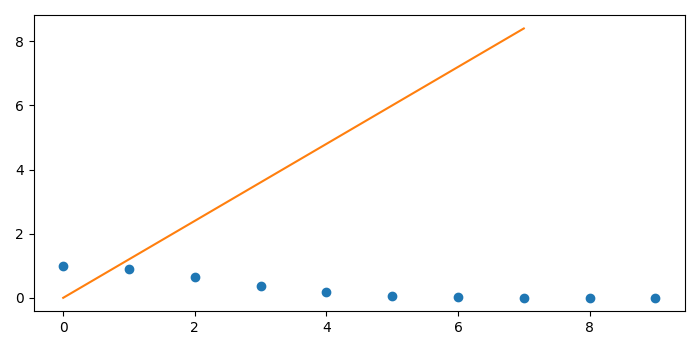# Python â€“ scipy.interpolate.interp1d

The interp1d() function of scipy.interpolate package is used to interpolate a 1-D function. It takes arrays of values such as x and y to approximate some function y = f(x) and then uses interpolation to find the value of new points.

## Syntax

scipy.interpolate.interp1d(x, y)

where x is a 1-D array of real values and y is an N-D array of real values. The length of y along the interpolation axis must be equal to the length of x.

## Example 1

Let us consider the following example −

# Import the required libraries
import matplotlib.pyplot as plt
import numpy as np
from scipy import interpolate

# Set the figure size
plt.rcParams["figure.figsize"]=[7.00, 3.50]
plt.rcParams["figure.autolayout"]=True

# Define the values
x = np.arange(0, 10)
y = np.exp(-x/5.0)

# Input Data
plt.subplot(1,2,1)
plt.title("Input X and Y")
plt.plot(x,y)

# Interpolated Data
plt.subplot(1,2,2)
plt.title("Interpolated")
f = interpolate.interp1d(x, y)
x_new = np.arange(0, 7, 0.7)
y_new = f(x_new)
plt.plot(x_new, y_new, 's')

plt.show()

## Output

The above program will generate the following output −## Example 2

Let us take another example −

# Import the required libraries
import matplotlib.pyplot as plt
import numpy as np
from scipy import interpolate

# Set the figure size
plt.rcParams["figure.figsize"]=[7.00, 3.50]
plt.rcParams["figure.autolayout"]=True

# Define the values
x = np.arange(0, 10)
y = np.exp(-x **2/9.0)

# interpolate function
f = interpolate.interp1d(x, y)
xnew = np.arange(0, 9, 1.2)
plt.plot(x, y, 'o', xnew)

plt.show()

## Output

The above program will generate the following output −Updated on: 24-Dec-2021

3K+ Views